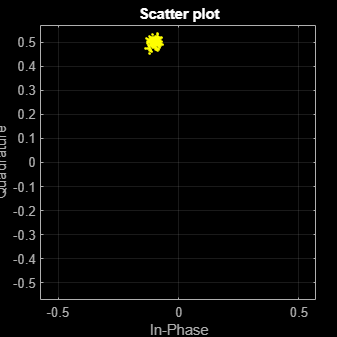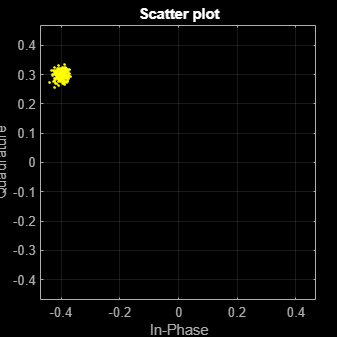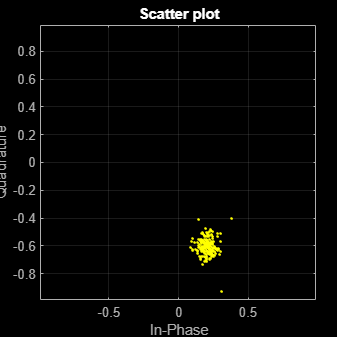# wlanLLTFChannelEstimate

Channel estimation using L-LTF

## Syntax

``chEst = wlanLLTFChannelEstimate(demodSig,cfg)``
``chEst = wlanLLTFChannelEstimate(demodSig,cbw)``
``chEst = wlanLLTFChannelEstimate(___,span)``

## Description

example

````chEst = wlanLLTFChannelEstimate(demodSig,cfg)` returns the channel estimate between the transmitter and all receive antennas using the demodulated L-LTF1 , `demodSig`, given the parameters specified in configuration object `cfg`. ```

example

````chEst = wlanLLTFChannelEstimate(demodSig,cbw)` returns the channel estimate given channel bandwidth `cbw`. The channel bandwidth can be used instead of the configuration object.```

example

````chEst = wlanLLTFChannelEstimate(___,span)` returns the channel estimate and performs frequency smoothing over the specified filter span. For more information, see Frequency Smoothing.This syntax supports input options from prior syntaxes. ```

## Examples

collapse all

Create VHT format configuration object. Generate a time-domain waveform for an 802.11ac VHT packet.

```vht = wlanVHTConfig; txWaveform = wlanWaveformGenerator([1;0;0;1],vht);```

Multiply the transmitted VHT signal by -0.1 + 0.5i and pass it through an AWGN channel with a 30 dB signal-to-noise ratio.

`rxWaveform = awgn(txWaveform*(-0.1+0.5i),30);`

Extract the L-LTF field indices and demodulate the L-LTF. Perform channel estimation without frequency smoothing.

```idxLLTF = wlanFieldIndices(vht,'L-LTF'); demodSig = wlanLLTFDemodulate(rxWaveform(idxLLTF(1):idxLLTF(2),:),vht); est = wlanLLTFChannelEstimate(demodSig,vht);```

Plot the channel estimate.

```scatterplot(est) grid```The channel estimate matches the complex channel multiplier.

Generate a time domain waveform for an 802.11n HT packet, pass it through a TGn fading channel and perform L-LTF channel estimation. Trailing zeros are added to the waveform to allow for TGn channel delay.

Create the HT packet configuration and transmit waveform.

```cfgHT = wlanHTConfig; txWaveform = wlanWaveformGenerator([1;0;0;1],cfgHT);```

Configure a TGn channel with 20 MHz bandwidth.

```tgnChannel = wlanTGnChannel; tgnChannel.SampleRate = 20e6;```

Pass the waveform through the TGn channel, adding trailing zeros to allow for channel delay.

`rxWaveform = tgnChannel([txWaveform; zeros(15,1)]);`

Skip the first four samples to synchronize the received waveform for channel delay.

`rxWaveform = rxWaveform(5:end,:);`

Extract the L-LTF and perform channel estimation.

```idnLLTF = wlanFieldIndices(cfgHT,'L-LTF'); sym = wlanLLTFDemodulate(rxWaveform(idnLLTF(1):idnLLTF(2),:),cfgHT); est = wlanLLTFChannelEstimate(sym,cfgHT);```

Create a VHT format configuration object. Using these objects, generate a time-domain waveform for an 802.11ac VHT packet.

```vht = wlanVHTConfig('ChannelBandwidth','CBW80'); txWaveform = wlanWaveformGenerator([1;0;0;1],vht);```

Multiply the transmitted VHT signal by -0.4 + 0.3i and pass it through an AWGN channel.

`rxWaveform = awgn(txWaveform*(-0.4+0.3i),30);`

Specify the channel bandwidth for demodulation and channel estimation. Extract the L-LTF field indices, demodulate the L-LTF, and perform channel estimation without frequency smoothing.

```chanBW = 'CBW80'; idxLLTF = wlanFieldIndices(vht,'L-LTF'); demodSig = wlanLLTFDemodulate(rxWaveform(idxLLTF(1):idxLLTF(2),:),chanBW); est = wlanLLTFChannelEstimate(demodSig,chanBW);```

Plot the channel estimate.

```scatterplot(est) grid```The channel estimate matches the complex channel multiplier.

Create a VHT format configuration object. Generate a time-domain waveform for an 802.11ac VHT packet.

```vht = wlanVHTConfig; txWaveform = wlanWaveformGenerator([1;0;0;1],vht);```

Multiply the transmitted VHT signal by 0.2 - 0.6i and pass it through an AWGN channel having a 10 dB SNR.

`rxWaveform = awgn(txWaveform*complex(0.2,-0.6),10);`

Extract the L-LTF from the received waveform. Demodulate the L-LTF.

```idxLLTF = wlanFieldIndices(vht, 'L-LTF'); lltfDemodSig = wlanLLTFDemodulate(rxWaveform(idxLLTF(1):idxLLTF(2),:),vht);```

Use the demodulated L-LTF signal to generate the channel estimate.

`est = wlanLLTFChannelEstimate(lltfDemodSig,vht);`

Plot the channel estimate.

```scatterplot(est) grid```The channel estimate is noisy, which may lead to inaccurate data recovery.

Estimate the channel again with the filter span set to 11.

```est = wlanLLTFChannelEstimate(lltfDemodSig,vht,11); scatterplot(est) grid```The filtering provides a better channel estimate.

Create a VHT format configuration object. Generate L-LTF and VHT-SIG-A fields.

```vht = wlanVHTConfig; txLLTF = wlanLLTF(vht); txSig = wlanVHTSIGA(vht);```

Create a TGac channel for an 80 MHz bandwidth and a Model-A delay profile. Pass the transmitted L-LTF and VHT-SIG-A signals through the channel.

```tgacChan = wlanTGacChannel('SampleRate',80e6,'ChannelBandwidth','CBW80', ... 'DelayProfile','Model-A'); rxLLTFNoNoise = tgacChan(txLLTF); rxSigNoNoise = tgacChan(txSig);```

Create an AWGN noise channel with an SNR = 15 dB. Add the AWGN noise to L-LTF and VHT-SIG-A signals.

```chNoise = comm.AWGNChannel('NoiseMethod','Signal to noise ratio (SNR)', ... 'SNR',15); rxLLTF = chNoise(rxLLTFNoNoise); rxSig = chNoise(rxSigNoNoise);```

Create an AWGN channel having a noise variance corresponding to a 9 dB noise figure receiver. Pass the faded signals through the AWGN channel.

```nVar = 10^((-228.6 + 10*log10(290) + 10*log10(80e6) + 9)/10); awgnChan = comm.AWGNChannel('NoiseMethod','Variance','Variance',nVar); rxLLTF = awgnChan(rxLLTF); rxSig = awgnChan(rxSig);```

`demodLLTF = wlanLLTFDemodulate(rxLLTF,vht);`

Estimate the channel using the demodulated L-LTF.

`chEst = wlanLLTFChannelEstimate(demodLLTF,vht);`

Recover the VHT-SIG-A signal and verify that there was no CRC failure.

```[recBits,crcFail] = wlanVHTSIGARecover(rxSig,chEst,nVar,'CBW80'); crcFail```
```crcFail = logical 0 ```

## Input Arguments

collapse all

Demodulated L-LTF OFDM symbols, specified as an NST-by-NSYM-by-NR array. NST is the number of occupied subcarriers. NSYM is the number of demodulated L-LTF symbols (one or two). NR is the number of receive antennas. Each column of the 3-D array is a demodulated L-LTF OFDM symbol. If you specify two L-LTF symbols, `wlanLLTFChannelEstimate` averages the channel estimate over both symbols.

Data Types: `single` | `double`
Complex Number Support: Yes

Format configuration, specified as one of these objects:

The `wlanLLTFChannelEstimate` function uses the `ChannelBandwidth` property of `cfg`.

Channel bandwidth, specified as one of these values.

• `'CBW5'` — Channel bandwidth of 5 MHz

• `'CBW10'` — Channel bandwidth of 10 MHz

• `'CBW20'` — Channel bandwidth of 20 MHz

• `'CBW40'` — Channel bandwidth of 40 MHz

• `'CBW80'` — Channel bandwidth of 80 MHz

• `'CBW160'` — Channel bandwidth of 160 MHz

• `'CBW320'` — Channel bandwidth of 320 MHz

Data Types: `char` | `string`

Filter span of the frequency smoothing filter, specified as a positive odd integer and expressed as a number of subcarriers. Frequency smoothing is applied only when `span` is specified and is greater than one. See Frequency Smoothing.

Data Types: `single` | `double`

## Output Arguments

collapse all

Channel estimate containing data and pilot subcarriers, returned as an NST-by-1-by-NR array. NST is the number of occupied subcarriers. The value of 1 corresponds to the single transmitted stream in the L-LTF. NR is the number of receive antennas.

collapse all

### L-LTF

The L-LTF is the second field in the 802.11™ OFDM PLCP legacy preamble. The L-LTF is a component of EHT, HE, VHT, HT, and non-HT PPDUs.Channel estimation, fine frequency offset estimation, and fine symbol timing offset estimation rely on the L-LTF.

The L-LTF is composed of a cyclic prefix (CP) followed by two identical long training symbols (C1 and C2). The CP consists of the second half of the long training symbol.The L-LTF duration varies with channel bandwidth.

Channel Bandwidth (MHz)Subcarrier Frequency Spacing ΔF (kHz)Fast Fourier Transform (FFT) Period (TFFT = 1 / ΔF)Cyclic Prefix or Training Symbol Guard Interval (GI2) Duration (TGI2 = TFFT / 2)L-LTF Duration (TLONG = TGI2 + 2 × TFFT)
20, 40, 80, 160, and 320312.53.2 μs1.6 μs8 μs
10156.256.4 μs3.2 μs16 μs
578.12512.8 μs6.4 μs32 μs

### Frequency Smoothing

Frequency smoothing can improve channel estimation by averaging out noise.

Frequency smoothing is recommended only for cases in which a single transmit antenna is used. Frequency smoothing consists of applying a moving-average filter that spans multiple adjacent subcarriers. Channel conditions dictate whether frequency smoothing is beneficial.

• If adjacent subcarriers are highly correlated, frequency smoothing results in significant noise reduction.

• In a highly frequency-selective channel, smoothing can degrade the quality of the channel estimate.

 Van de Beek, J.-J., O. Edfors, M. Sandell, S. K. Wilson, and P. O. Borjesson. “On Channel Estimation in OFDM Systems.” Vehicular Technology Conference, IEEE 45th, Volume 2, IEEE, 1995.

 IEEE Std 802.11-2020 (Revision of IEEE Std 802.11-2016). “Part 11: Wireless LAN Medium Access Control (MAC) and Physical Layer (PHY) Specifications.” IEEE Standard for Information Technology — Telecommunications and Information Exchange between Systems — Local and Metropolitan Area Networks — Specific Requirements.